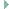periph_cpu.h File Reference

CPU specific definitions and functions for peripheral handling. More...

## Detailed Description

CPU specific definitions and functions for peripheral handling.

Definition in file periph_cpu.h.

`#include <stdint.h>`
`#include <limits.h>`
`#include "eagle_soc.h"`
`#include "cpu_conf.h"`Include dependency graph for periph_cpu.h:

Go to the source code of this file.

## Data Structures

struct  i2c_conf_t
I2C configuration structure. More...

struct  spi_conf_t
SPI device configuration. More...

struct  uart_conf_t
UART device configuration. More...

## Macros

#define GPIO_UNDEF   ((gpio_t)(UINT_MAX))
Definition of a fitting UNDEF value.

#define GPIO_PIN(x, y)   ((x & 0) | y)
Define a CPU specific GPIO pin generator macro.

#define PORT_GPIO   (0)
Available GPIO ports on ESP8266.

#define GPIO_PIN_NUMOF   (17)
Define CPU specific number of GPIO pins.

#define PERIPH_TIMER_PROVIDES_SET
Prevent shared timer functions from being used.

#define CPUID_LEN   (4U)
Length of the CPU_ID in octets.

## GPIO configuration

#define HAVE_GPIO_T
Override the default gpio_t type definition. More...

typedef unsigned int gpio_t

## Predefined GPIO names

#define GPIO0   (GPIO_PIN(PORT_GPIO,0))

#define GPIO1   (GPIO_PIN(PORT_GPIO,1))

#define GPIO2   (GPIO_PIN(PORT_GPIO,2))

#define GPIO3   (GPIO_PIN(PORT_GPIO,3))

#define GPIO4   (GPIO_PIN(PORT_GPIO,4))

#define GPIO5   (GPIO_PIN(PORT_GPIO,5))

#define GPIO6   (GPIO_PIN(PORT_GPIO,6))

#define GPIO7   (GPIO_PIN(PORT_GPIO,7))

#define GPIO8   (GPIO_PIN(PORT_GPIO,8))

#define GPIO9   (GPIO_PIN(PORT_GPIO,9))

#define GPIO10   (GPIO_PIN(PORT_GPIO,10))

#define GPIO11   (GPIO_PIN(PORT_GPIO,11))

#define GPIO12   (GPIO_PIN(PORT_GPIO,12))

#define GPIO13   (GPIO_PIN(PORT_GPIO,13))

#define GPIO14   (GPIO_PIN(PORT_GPIO,14))

#define GPIO15   (GPIO_PIN(PORT_GPIO,15))

#define GPIO16   (GPIO_PIN(PORT_GPIO,16))

## I2C configuration

ESP8266 provides up to two bit-banging I2C interfaces.

The board-specific configuration of the I2C interface I2C_DEV(n) requires the definition of

I2Cn_SPEED, the bus speed, I2Cn_SCL, the GPIO used as SCL signal, and I2Cn_SDA, the GPIO used as SDA signal,

where n can be 0 or 1. If they are not defined, the I2C interface I2C_DEV(n) is not used.

Note
The configuration of the I2C interfaces I2C_DEV(n) must be in continuous ascending order of n.

I2C_NUMOF is determined automatically from board-specific peripheral definitions of I2Cn_SPEED, I2Cn_SCK, and I2Cn_SDA.

#define I2C_NUMOF_MAX   (2)
Maximum number of I2C interfaces that can be used by board definitions.

#define PERIPH_I2C_NEED_WRITE_REG
i2c_write_reg required

#define PERIPH_I2C_NEED_WRITE_REGS
i2c_write_regs required

## PWM configuration

The hardware implementation of ESP8266 PWM supports only frequencies as power of two.

Therefore a software implementation of one PWM device PWM_DEV(0) with up to 8 PWM channels (PWM_CHANNEL_NUM_MAX) is used. The GPIOs that can be used as PWM channels are defined by PWM0_GPIOS in board definition.

Note
The minimum PWM period that can be realized is 10 us or 100.000 PWM clock cycles per second. Therefore, the product of frequency and resolution should not be greater than 100.000. Otherwise the frequency is scaled down automatically.
#define PWM_NUMOF_MAX   (1)
Maximum number of PWM devices.

#define PWM_CHANNEL_NUM_MAX   (8)
Maximum number of channels per PWM device.

## RNG configuration

The address of the register for accessing the hardware RNG.

## RTT and RTC configuration

#define RTT_FREQUENCY   (312500UL)

#define RTT_MAX_VALUE   (0xFFFFFFFFUL)

## SPI configuration

ESP8266 has two SPI controllers:

• CSPI for caching and accessing the flash memory
• HSPI for peripherals

Thus, HSPI is the only SPI interface that is available for peripherals. It is exposed as RIOT's SPI_DEV(0). The pin configuration of the HSPI interface is fixed as shown in following table.

Signal Pin
SPI0_MISO GPIO12
SPI0_MOSI GPIO13
SPI0_SCK GPIO14
SPI0_CS0 GPIOn with n = 0, 2, 4, 5, 15, 16 (additionally 9, 10 in DOUT flash mode)

The only pin definition that can be overridden by an application-specific board configuration is the CS signal defined by SPI0_CS0.

#define SPI_NUMOF_MAX   (1)
Maximum number of SPI interfaces.

#define PERIPH_SPI_NEEDS_TRANSFER_BYTE
requires function spi_transfer_byte

#define PERIPH_SPI_NEEDS_TRANSFER_REG
requires function spi_transfer_reg

#define PERIPH_SPI_NEEDS_TRANSFER_REGS
requires function spi_transfer_regs

enum  spi_ctrl_t { HSPI = 1 }
SPI controllers that can be used for peripheral interfaces. More...

## UART configuration

All ESP8266 boards have exactly one UART device with fixed pin mapping.

#define UART_NUMOF_MAX   (2)
Maximum number of UART interfaces.

## ◆ HAVE_GPIO_T

 #define HAVE_GPIO_T

Override the default gpio_t type definition.

This is required here to have gpio_t defined in this file.

Definition at line 48 of file periph_cpu.h.

## ◆ spi_ctrl_t

 enum spi_ctrl_t

SPI controllers that can be used for peripheral interfaces.

Enumerator
HSPI

HSPI interface controller.

Definition at line 258 of file periph_cpu.h.